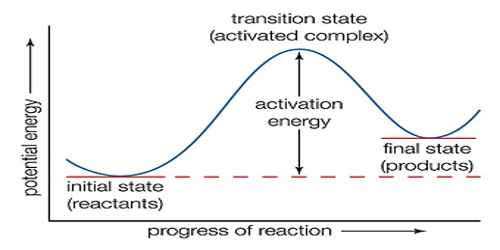# Theory of Absolute Reaction Rate

Theory of Absolute Reaction Rate

The theoretical approach to the calculation of the rates of reaction has been made from a point of view totally different from the collision theory. In the theory of absolute reaction rate or the transition-state theory which was developed by Eyring, Glasstone and Laidler, attention is focused on the activated complex. The activated complex is supposed to be in equilibrium with the reactants. According to absolute rate theory, the reaction between A and B may be represented by

A + B ↔ A … B → products

where A … B is the activated complex. The equilibrium constant for the formation of the complex is-

K = [A — B] / [A][B]

The concentration of the activated complex;

[A — B] = K [A][B]

In spite of its ill-defined character the activated complex is regarded as a molecule except that it has one special mode of vibration with respect to which it is unstable. This vibration may be regarded as having a large enough frequency which leads to dissociation of the complex into the products. The rate of the reaction is then the product at the concentration of the activated complex and the frequency of this vibration, v, i.e.,

rate = v [A…B] = v K [A][B]

But the rate of the reaction, A + B → — Products is

Rate = K [A][B]